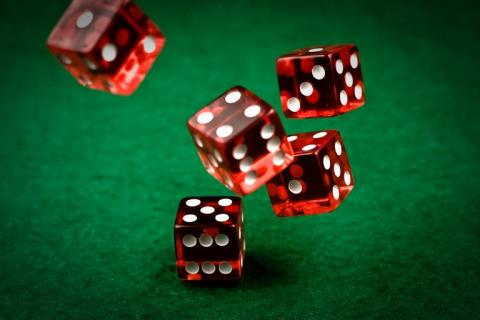# SC 2019 Semilab: Probability Theory

by Aleksei BorodinIf you toss three fair coins, what is the probability they all show the same, either all Heads or all Tails? Here is an argument from a Victorian scholar: "At least two of three coins must be alike. The third coin is equally likely to fall Head or Tails, so half the time it the same as the other two. Hence, the chance all three are the same is one-half." Is this a valid argument? Can one run an experiment and be sure that it yields the correct answer?

We will analyze many similar situations with coins and dice, play simple casino games (can you bankrupt a casino?), and use the theory of probability to choose winning sides.

Back to the list of semilabs